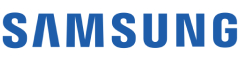New update is available. Click here to update.
Topics

# Insert Interval

Moderate0/80
Average time to solve is 30m+1 more companies

## Problem statement

You are given a 2-dimensional array ‘Intervals’ containing a list of non-overlapping intervals sorted by their start time. You are given an interval ‘newInterval’. Your task is to insert the given interval at the correct position and merge all necessary intervals to produce a list with only mutually exclusive intervals.

For Example:
``````Consider 'Intervals' = [[1, 3], [5, 7], [8, 12]], and 'newInterval' = [4, 6]
The interval [4, 6] overlaps with [5, 7]. Therefore we can merge the intervals and produce an interval [4, 7]. Hence the answer [[1,3], [4,7], [8,12]]
``````
Detailed explanation ( Input/output format, Notes, Images )
Constraints:
``````1 <= T <= 5
0 <= N <= 10^5
0 <= Intervals[i], Intervals[i] <= 10^10
0 <= newInterval, newInterval <= 10^10

Time Limit: 1 sec
``````
Sample Input 1:
``````2
3
1 3
5 7
8 12
4 6
3
1 3
5 7
8 12
4 10
``````
Sample Output 1:
``````1 3
4 7
8 12
1 3
4 12
``````
Explanation:
``````For the first test case,
The interval [4, 6] overlaps with [5, 7]. Therefore we can merge the intervals and produce an interval [4, 7]. Hence the answer [[1,3], [4,7], [8,12]].

For the second test case,
The interval [4, 10] overlaps with [5, 7] and [8, 12]. Therefore we can merge the intervals and produce an interval [4, 12]. Hence the answer is [[1, 3], [4, 12]].
``````
Sample Input 2:
``````2
2
2 3
5 7
1 4
2
1 2
6 9
3 5
``````
Sample Output 2:
``````1 4
5 7
1 2
3 5
6 9
``````Console